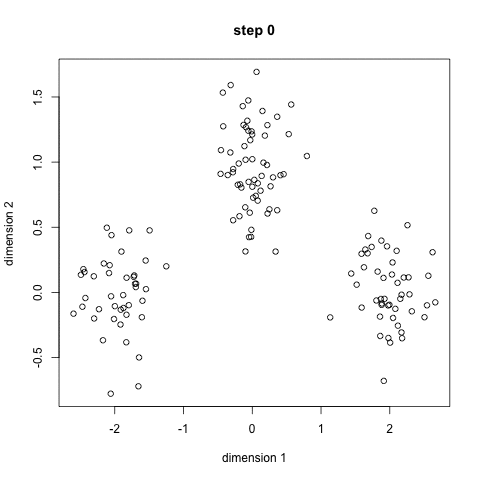# K-Means

K-Means is probably the most well know clustering algorithm. It’s taught in a lot of introductory data science and machine learning classes. It’s easy to understand and implement in code! Check out the graphic below for an illustration. K-Means has the advantage that it’s pretty fast, as all we’re really doing is computing the distances between points and group centers; very few computations! It thus has a linear complexity O(n). On the other hand, K-Means has a couple of disadvantages. Firstly, you have to select how many groups/classes there are. This isn’t always trivial and ideally with a clustering algorithm we’d want it to figure those out for us because the point of it is to gain some insight from the data. K-means also starts with a random choice of cluster centers and therefore it may yield different clustering results on different runs of the algorithm. Thus, the results may not be repeatable and lack consistency. Other cluster methods are more consistent.

Overview of algorithm:

1. Choose the number of k clusters
2. Randomly assign items to the k clusters
3. Calculate new centroid for each of the k clusters
4. Calculate the distance of all items to the k centroids
5. Assign items to closest centroid
6. Repeat until clusters assignments are stable

K-Medians is another clustering algorithm related to K-Means, except instead of recomputing the group center points using the mean we use the median vector of the group. This method is less sensitive to outliers (because of using the Median) but is much slower for larger datasets as sorting is required on each iteration when computing the Median vector. The 5 Clustering Algorithms Data Scientists Need to Know | Towards Data ScienceThe algorithm follows a procedure to form clusters which contain homogeneous data points. The value of k is an input for the algorithm. Based on that, algorithm selects k number of centroids. Then the neighboring data points to a centroid combines with its centroid and creates a cluster. Later a new centroid is created within each cluster. Then data points near to new centroid will combine again to expand the cluster. This process is continued until centroids do not change. 10 Machine Learning Algorithms You need to Know | Sidath Asir @ Medium